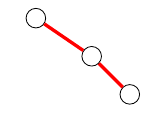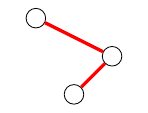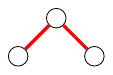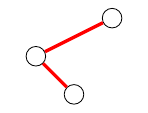Queries for Binary trees: search statistic / browse statistics / browse maps from / browse maps to

# 1. Definition & Example

• A binary tree is a rooted tree where each node is either internal (has two children) or is a leaf (has no children).

• Equivalently, a binary tree is recursively defined to be either an empty tree (leaf) or an ordered pair of binary trees (internal node).

 the 5 Binary trees of size 3[.,[.,[.,.]]] [.,[[.,.],.]] [[.,.],[.,.]] [[.,[.,.]],.] [[[.,.],.],.]
• The graphical representation omits the leafs and only shows the internal nodes.
• There are $\operatorname{Cat}(n) = \frac{1}{n+1}\binom{2n}{n}$ binary trees with $n$ internal nodes, see OEIS:A000108.

# 2. Additional information

Feel free to add further information on the combinatorics of binary trees here!

# 5. Technical information for database usage

• A binary tree is uniquely represented as a dot (empty tree) or as a sorted list of binary trees.

• Binary trees are graded by the number of internal nodes.

• The database contains all binary trees of size at most 8.